Test: Data Handling

# Test: Data Handling

Test Description

## 20 Questions MCQ Test Mathematics for Class 5: NCERT | Test: Data Handling

Test: Data Handling for Class 5 2022 is part of Mathematics for Class 5: NCERT preparation. The Test: Data Handling questions and answers have been prepared according to the Class 5 exam syllabus.The Test: Data Handling MCQs are made for Class 5 2022 Exam. Find important definitions, questions, notes, meanings, examples, exercises, MCQs and online tests for Test: Data Handling below.
Solutions of Test: Data Handling questions in English are available as part of our Mathematics for Class 5: NCERT for Class 5 & Test: Data Handling solutions in Hindi for Mathematics for Class 5: NCERT course. Download more important topics, notes, lectures and mock test series for Class 5 Exam by signing up for free. Attempt Test: Data Handling | 20 questions in 20 minutes | Mock test for Class 5 preparation | Free important questions MCQ to study Mathematics for Class 5: NCERT for Class 5 Exam | Download free PDF with solutions
 1 Crore+ students have signed up on EduRev. Have you?
Test: Data Handling - Question 1

### Direction: The given bar graph shows the number of residents in 6 cities. Study the graph and answer the question.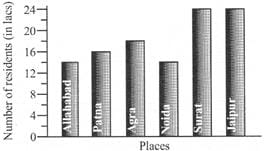Q. There were 18 lacs residents residing in ____.

Test: Data Handling - Question 2

### Direction: The given bar graph shows the number of residents in 6 cities. Study the graph and answer the question.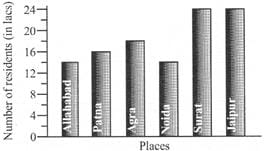Q. How many more residents were residing in Surat than in Agra?

Test: Data Handling - Question 3

### Direction: The given bar graph shows the number of residents in 6 cities. Study the graph and answer the question.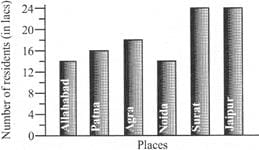Q. How many residents were there altogether in the 6 cities?

Test: Data Handling - Question 4

Direction: The given bar graph shows the number of viewers who watched the 4 channels. Study the graph and answer the question.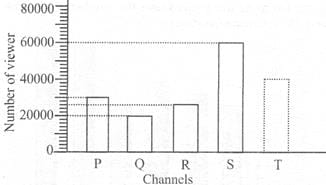Q. How many more viewers watched Channel S than Channel Q?

Test: Data Handling - Question 5

Direction: The given bar graph shows the number of viewers who watched the 4 channels. Study the graph and answer the question.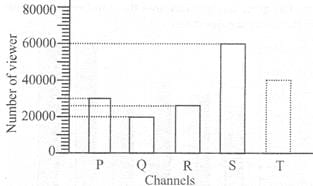Q. There were 150000 viewers altogether. How many viewers watched Channel T?

Test: Data Handling - Question 6

Direction: The Sine graph shows the quantity of petrol Ankit used and the distance his car travelled. Study the graph answer the question.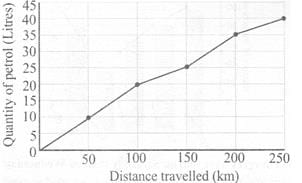Q. How much less petrol was used for 100 km 200 km travelled?

Test: Data Handling - Question 7

Direction: The Sine graph shows the quantity of petrol Ankit used and the distance his car travelled. Study the graph answer the question.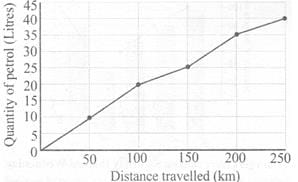Q. What distance was travelled with 20 litres of petrol?

Test: Data Handling - Question 8

Direction: The Sine graph shows the quantity of petrol Ankit used and the distance his car travelled. Study the graph answer the question.Q. What distance was travelled with 35 litres of petrol?

Test: Data Handling - Question 9

Direction: Study the graph and answer the question.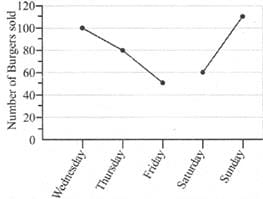Q. How many less burgers were sold on Saturday than on Wednesday?

Test: Data Handling - Question 10

Direction: Study the graph and answer the question.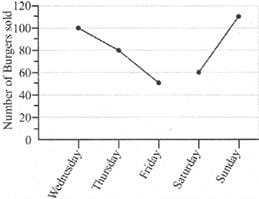Q. The sum of the greatest number of burgers and the smallest number burgers sold is

Test: Data Handling - Question 11

Direction: Study the graph and answer the question.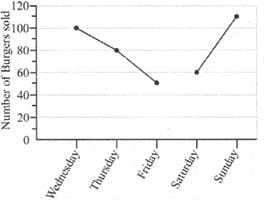Q. If a profit of Rs. 12 was made for every burger sold, then how much profit was made on Thursday?

Test: Data Handling - Question 12

Direction: The bar graph shows the mass of 5 children.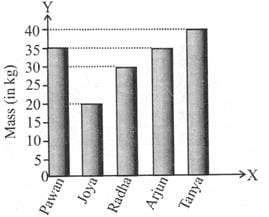Q. What is the difference between the mass of the heaviest child and the lightest child?

Detailed Solution for Test: Data Handling - Question 12

Mass of the heaviest child = 40 kg Mass of the lightest child = 20 kg Their difference = 20 kg

Test: Data Handling - Question 13

Direction: The bar graph shows the mass of 5 children.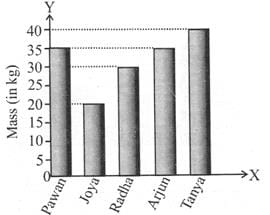Q. Which of the two children have the same mass?

Detailed Solution for Test: Data Handling - Question 13

From the graph, the two bars corresponding to the mass of Pawan and Arjun are of the same height. So, Pawan and Arjun have the same mass.

Test: Data Handling - Question 14

Direction: The bar graph shows the mass of 5 children.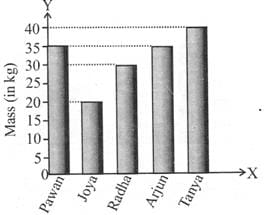Q. Who is 20 kg heavier than Joya?

Detailed Solution for Test: Data Handling - Question 14

Mass of Joya = 20 kg 20 kg heavier than Joya = 20 kg + 20 kg = 40 kg = Mass of Tanya

Test: Data Handling - Question 15

Direction: The graph shows how Lalit spends his pocket money each month.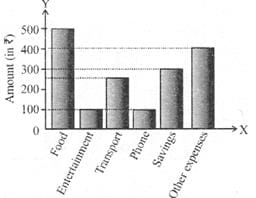Q. The amount spent on food is how many times the amount spent on transport?

Test: Data Handling - Question 16

Direction: The graph shows how Lalit spends his pocket money each month.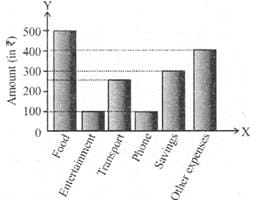Q. What is the fraction of the amount spent on other expenses to the amount spent on food?

Test: Data Handling - Question 17

Direction: The graph shows how Lalit spends his pocket money each month.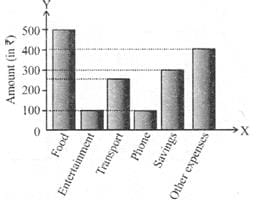Q. How much does Lalit earn per month?

Test: Data Handling - Question 18

The pie-chart shows the modes of transport used by students of a school. XY is a straight line and 0 is the centre of the circle. If 45 students go to school by taxi, how many of them go by scooter?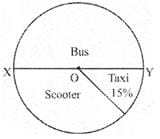Test: Data Handling - Question 19

Direction: The line graph shows the money earned by a fruit seller during a certain week.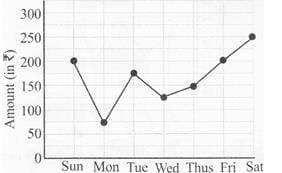Q. On which day did he earn Rs.75 less than the amount earned on Thursday?

Detailed Solution for Test: Data Handling - Question 19

Amount earned on Thursday = Rs. 150

∴ Rs. 75 less than Rs.150 is Rs. 75 = Amount earned on Monday

Test: Data Handling - Question 20

Direction: The line graph shows the money earned by a fruit seller during a certain week.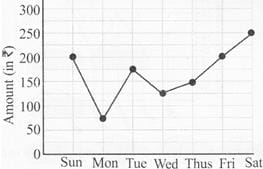Q. What is the average amount earned from Monday to Thursday?

## Mathematics for Class 5: NCERT

33 videos|59 docs|39 tests
 Use Code STAYHOME200 and get INR 200 additional OFF Use Coupon Code
Information about Test: Data Handling Page
In this test you can find the Exam questions for Test: Data Handling solved & explained in the simplest way possible. Besides giving Questions and answers for Test: Data Handling , EduRev gives you an ample number of Online tests for practice

## Mathematics for Class 5: NCERT

33 videos|59 docs|39 tests